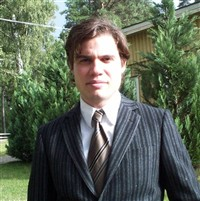# Welcome

 Welcome to my homepage! My name is Eric B Torbrand Dhrif and I am an independent researcher. My academic background is as follows; Masters or M.Sc. (Civilingenjör  Degree Engineering Physics)/ Mathematical Physics(Quantum Field Theory) Degree, highest academic distinction from KTH, Stockholm, Sweden.  A M Phil in Mathematical Finance from Uppsala University, Uppsala, Sweden. I live in Sweden and I work and have worked with  1) Mathematical Physics(Mostly QFT, Strings, Theoretical Quantum Gravity Physics, Analysis, Geometry). 2) Mathematical Finance(PDE's, Stochastic Analysis, Probability Theory, Martingales, Finance). My hobbies are cuisine, games and languages. This homepage is for communicating advances in, mostly, the areas of Mathematical Finance, Pure Mathematics and Mathematical Physics. My e-mail is eric.torbrand AT gmail.com   Keywords; Gravity, Quantum Gravity, Grand Unified Theory, Quantum Field Theory, Mathematical Physics, Mathematical Finance, String Theory, Analysis, Complex Geometry, Noncommutative Geometry.Me some years ago.